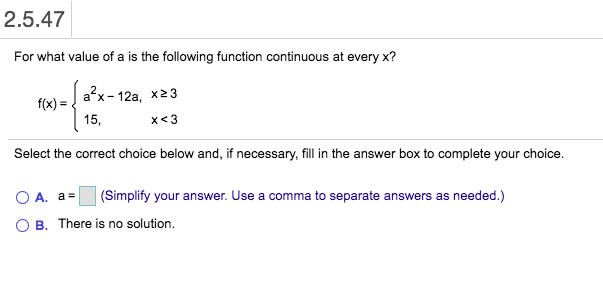# 2.5.47For what value of a is the following function continuous at every x?a x 12a, x23f(x)15,x

Question
1 views

Can I get help with this question step by step?help_outlineImage Transcriptionclose2.5.47 For what value of a is the following function continuous at every x? a x 12a, x23 f(x) 15, x<3 Select the correct choice below and, if necessary, fill in the answer box to complete your choice. (Simplify your answer. Use a comma to separate answers as needed.) A. a O B. There is no solution. fullscreen
check_circle

Step 1

Refer to the question,

The function provided is,

Step 2

For function to be continuous Left hand limit at that point should be equal to Right hand limit at that point which should be equal to function value at that point.

Step 3

Now use the above concept to...

### Want to see the full answer?

See Solution

#### Want to see this answer and more?

Solutions are written by subject experts who are available 24/7. Questions are typically answered within 1 hour.*

See Solution
*Response times may vary by subject and question.
Tagged in

### Calculus